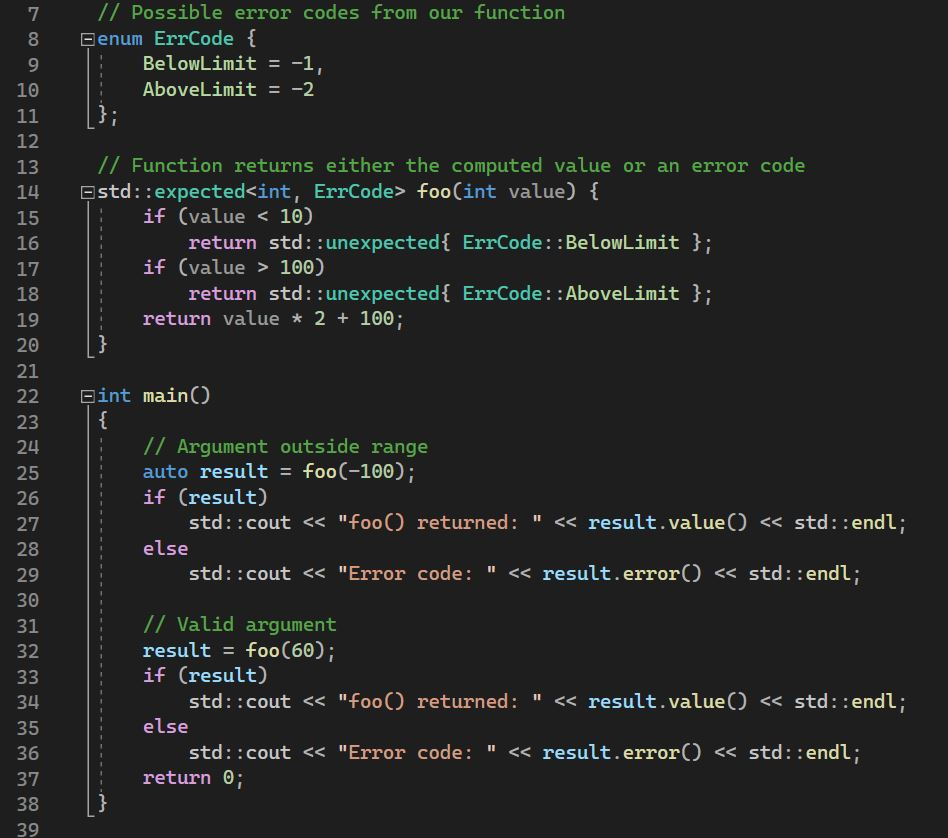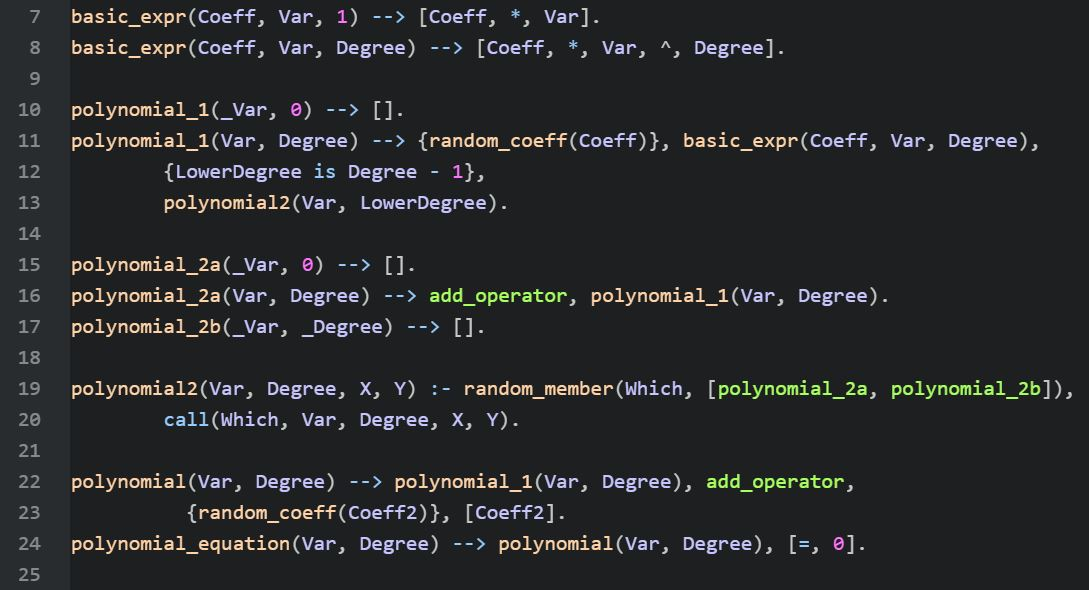Polynomial is an important topic in High School maths curriculum. There are many online courses that explain the topic in great details with lots of examples and sample exercises. Wouldn’t it be interesting if we can generate polynomials of given degree programmatically? It turns out that this is not such a hard problem after all. […]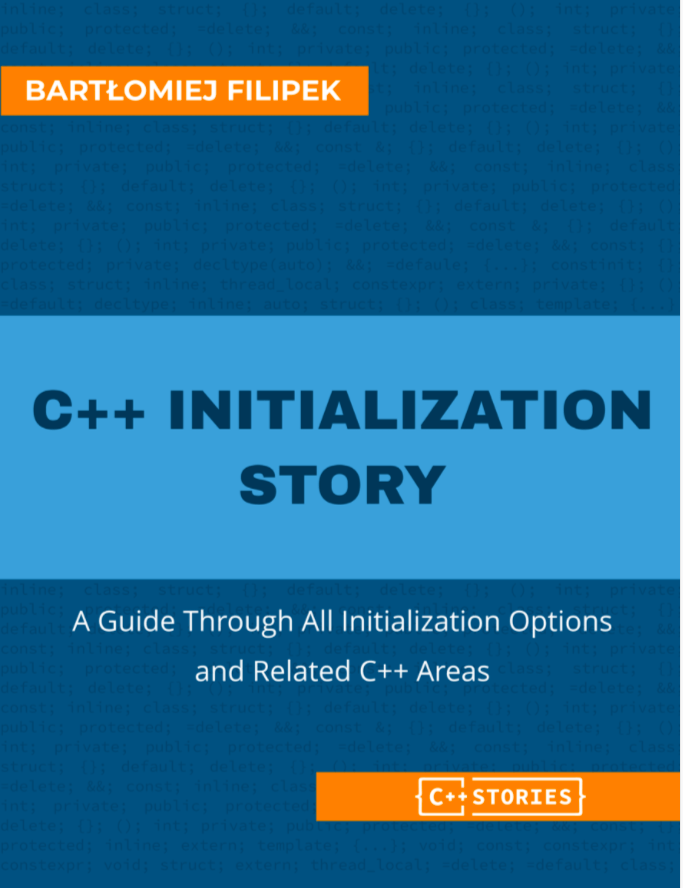Title: C++ Initialization Story – A Guide Through All Initialization Options and Related C++ Areas Author: Bartłomiej Filipek Publisher: Leanpub Year: 2023 Can you believe that there is a language where “initialization” itself involves many subtleties and a whole book can be devoted to this topic? Yes, modern C++ is such a language! While many […]The constinit specifier, introduced in C++20, is applied to static variables (global and local static) and thread local variables, with the requirement that they either have a zero initialization or they are initialized with a compile-time constant expression. Here is our first example: Line 17 declares a global constinit variable, initialized with a constant value. […]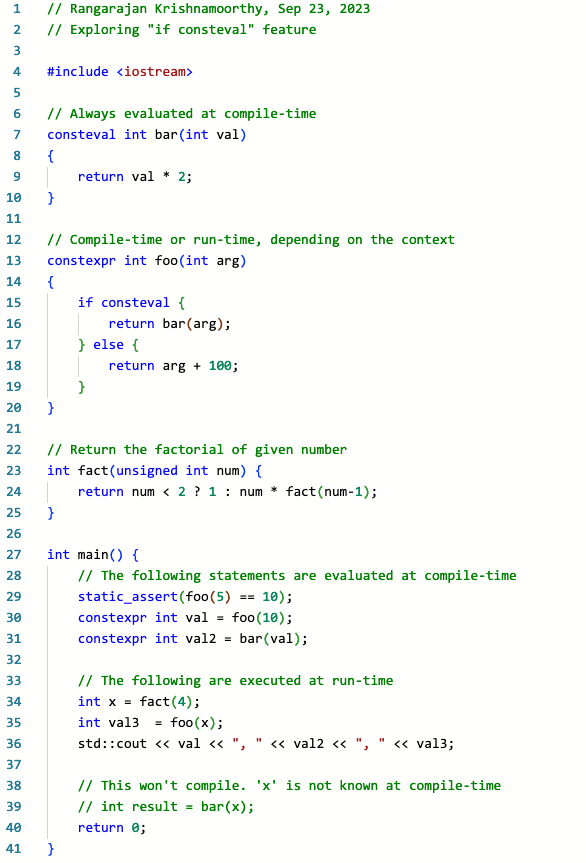“If consteval” is useful in the context of calling a consteval function from within a constexpr function. A consteval function can only be invoked in a constant expression and hence is evaluated at compile-time. On the other hand, a constexpr function may be invoked in a constant expression or non-constant expression. If invoked in a […]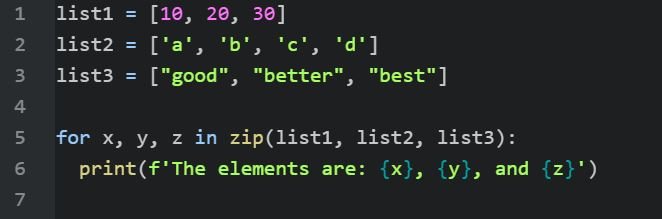The zip() function in Python is a convenient mechanism for iterating over multiple “iterables” in parallel. Looping over lists is a common scenario. Here is the output generated by the above code: Common Lisp does not have such a feature built into the language or as part of the standard library. Of course, we have […]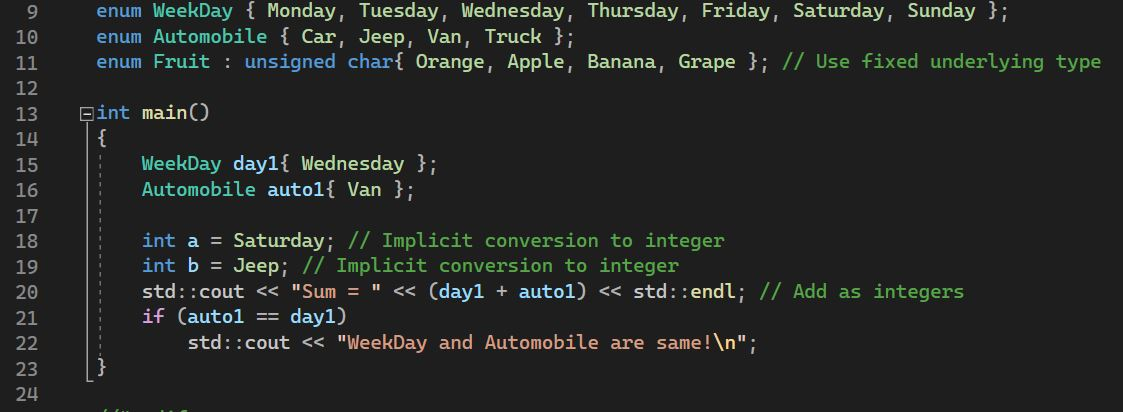The type trait “std::is_scoped_enum<T>::value” was introduced in C++23 to check whether the type “T” is a scoped enum type. Another way to use this is std::is_scoped_enum_v<T>. Before getting into this trait in detail, let us briefly recap the differences between unscoped and scoped enums. Unscoped Enums Unscoped enums are the old-style enums. Look at the […]In addition to the conventional “==” operator that checks if two objects are equal, Julia has the “===” operator to check for equality. What is the difference between these two operators? The “==” operator checks whether two objects have the same value. Here are some examples: Cell 5 shows that the RHS float value is […]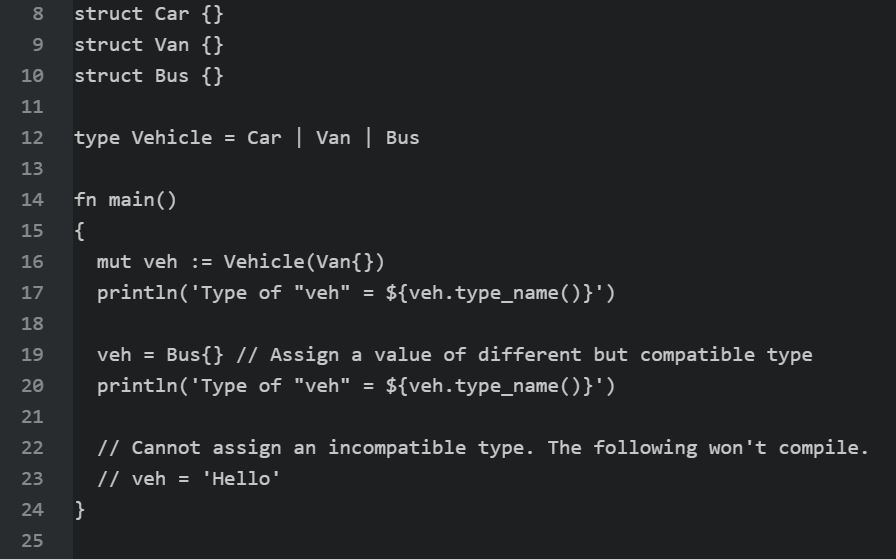A Sum data type in V language gives us a convenient way to hold objects of many distinct types (at any time, just one object) and hence is a discriminated union. For example, in C++ we have the variant type. Here is an example of sum type in V language: In the above program, the […]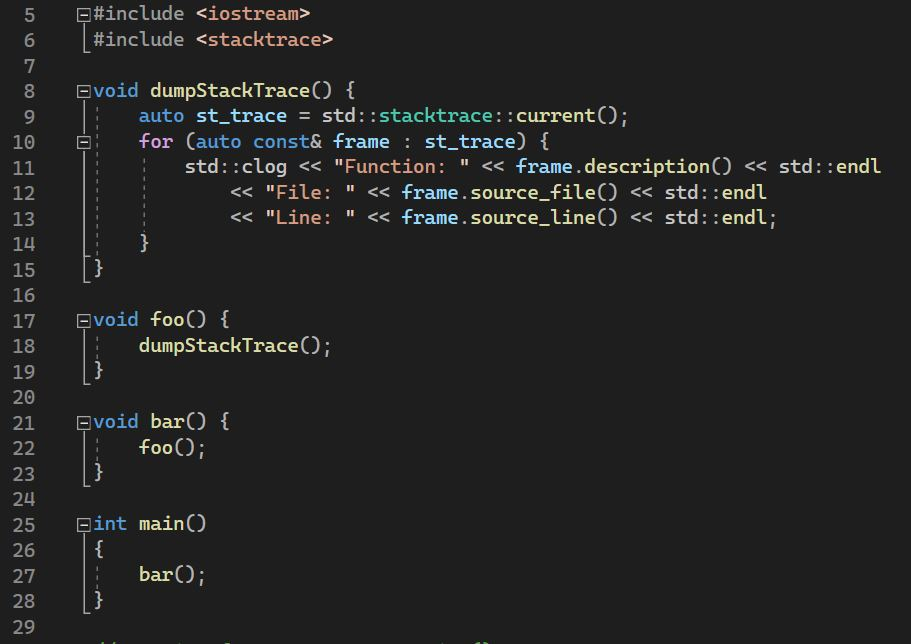The ability to enumerate stack frames at runtime is one of the interesting features introduced in C++23. This is made possible through the <stacktrace> header. The interface is quite simple. Here is a piece of code showing how to use the library. There are 4 functions and here is the call chain: The “dumpStackTrace()” function […]